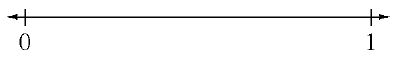### Home > CC1MN > Chapter 6 Unit 6 > Lesson CC1: 6.1.1 > Problem6-13

6-13.

Copy the number line and label the following numbers at their approximate place on the number line.1. $\frac { 14 } { 28 }$

1. $0.75$

1. $\frac { 1 } { 3 }$

1. $\frac { 4 } { 7 }$

$\text{Can you decide if each portion is greater than or less than }0.5\ (\text{or }\frac{1}{2})?$